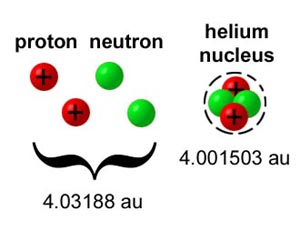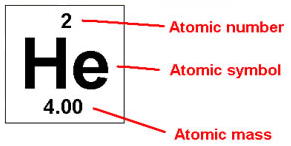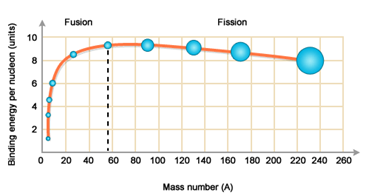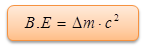×#### Thank you for registering.

One of our academic counsellors will contact you within 1 working day.

Click to Chat

1800-1023-196

+91-120-4616500

CART 0

• 0

MY CART (5)

Use Coupon: CART20 and get 20% off on all online Study Material

ITEM
DETAILS
MRP
DISCOUNT
FINAL PRICE
Total Price: Rs.

There are no items in this cart.
Continue Shopping• Complete Physics Course - Class 11
• OFFERED PRICE: Rs. 2,968
• View Details

Mass Defect and Binding Energy

Table of Content

Observation from the plot of B.E./ nucleons Vs mass number (A)

Related Resources

The rest mass of the stable nucleus of a stable atom is always less than the sum of the masses of constituent nucleons. The difference is called the mass defect Δm (i.e., Δm.c2) is utilised in keeping the nucleons bound together. This energy is known as the binding energy. In order to break the nucleus into its constituent nucleons an amount of energy equal to its binding energy has to be supplied to the nucleus. The mass defect per nucleon Δm/A = P, is called the packing fraction of the nucleus.Atomic mass is the mass of a single atomic particle or molecule. It is the sum of protons and electrons present in the atom of an element. It is expressed in mole. It is simply a collection of nuclides that make up a chemical element. it is a whole number.

Atomic weight is the ratio of atom of an element. The average weight of an atom is relative to the 1/12 weight of the carbon -12 atom. It is also referred to as relative atomic mass.The value is not necessarily a whole number.

Atomic weight = [Mass(a)isotope(a)]+[Mass(b)isotope(b)]The nucleons are bound together in a nucleus and the energy has to be supplied in order to break apart the constituents into free nucleons. The energy with which nucleons are bounded together in a nucleus is called as Binding Energy (B.E.). In order to free nucleons from a bounded nucleus this much of energy (= B.E.) is to be supplied.

It is observed that the mass of a nucleus is always less than the mass of constituent (free) nucleons. This difference in mass is called as mass defect and is denoted as Dm.

If mn: mass of a neutron;

mp: mass of a proton

M (Z, A): mass of bounded nucleus

Then, Δm = Z . mp + (A – Z). mn – M (Z, A)This mass-defect is in form of energy and is responsible for binding the nucleons together. From Einstein's law of inter-conversion of mass into energy:

E = mc2     (c: speed of light; m: mass)

Binding energy,Generally, Δm is measured in amu units. So let us calculate the energy equivalent to 1 amu. It is calculated in eV (electron volts; 1 eV =1.6 x10–19J)

E (= 1 amu = 1.67 × 10–27 (3×108)2 / 1.6 × 10–19) eV = 931 × 108 eV = 931 MeV

=> B.E. = Δm (931) MeV

There is another quantity which is very useful in predicting the stability of a nucleus called as Binding energy per nucleons.

B.E. per nucleons = Δm (931) / A MeV

Observation from the plot of B.E./nucleons Vs mass number (A):-

(i) B.E./nucleons increases on an average and reaches a maximum of about 8.7 MeV for A º 50 – 80.

(ii) For more heavy nuclei, B.E./nucleons decreases slowly as A increases. For the heaviest natural element U238 it drops to about 7.5 MeV.

(iii) From above observation, it follows that nuclei in the region of atomic masses 50-80 are most stable.

Refer this video for better understanding about mass defect and binding energy:-

Problem 1:-:

If mass of proton = 1.008 amu and mass of neutron = 1.009 amu, then the binding energy per nucleon for 4Be9 (mass = 9.012 amu) will be:

(A) 0.0672 MeV                (B) 0.672 MeV

(C) 6.72 MeV                   (D) 67.2 MeV

Solution:-

Mass defect,

Δm = (4 × 1.008 + 5 × 1.009) – 9.012

= 9.077 – 9.012 =0.065 amu

BE/A = 0.065 × 931 / 9 = 6.72 MeV

Problem 2:-:

The energy released in the following b-decay process will be:Given that,

mn = 1.6747 × 10–27 kg

mp = 1.6725 × 10–27 kg

me = 0.00091 × 10–27 kg

(A)    0.931 MeV                 (B)    0.731 MeV

(C)    0.511 MeV                 (D)    0.271 MeV

Solution:-

Mass defect Δm = (1.6747 – 1.6725 – 0.0091) × 10–27 = 0.0012 × 10–27 kg

ΔE = 0.0012 × 10–27 × (3 × 108)2 / 1.6 × 10–12 = 0.731 MeV

Problem 3:-:

If the mass of 3Li7 is 7.01653 amu, then find out binding energy per nucleon for 3Li7 .

(A)    5.6 MeV                     (B)    39.25 MeV

(C)    1 MeV                        (D)    zero.

Solution:-

E = ΔE / A = Δm × 931 / A MeV

Δm = (3mp + 4mn) – mass of Li7

= (3 × 1.00759 + 4 × 1.008898) – 7.01653

= 0.04216

ΔE = 0.04216 × 931 / 7 = 39.25 / 7 = 5.6 MeV

How much energy is released in the following reaction?

1H2 + 1H2 = 2He4

If the B.E./Nucleon of 1H2 and 2He4 are 1.123 MeV and 7.2 MeV respectively.

(A)    12 MeV                      (B)    24.3 MeV

(C)    36 MeV                      (D)    zero

Solution:-

B. E. of 1H2,

ΔE = 1.125

E = A × ΔE

E = 2 × 1.125

= 2.25 MeV

B.E. of two 1H2 = 2.25

Ed = 4.5 MeV

B.E. of an α -particle = 4 × 7.2

Ea = 28.8

Energy released ER = Ea – Ed

ER = 28.8 – 4.5 = 24.3 MeV

From the above observation we conclude that, option (B) is correct.The mass defect per nucleon is called packing fraction of the nucleus.

In order to break the nucleus into its constituent nucleons an amount of energy equal to this binding energy has to be supplied to the nucleus.

Rest mass of the stable nucleus of a stable atom is always less than the sum of the masses of constituent nucleons.

When a heavy nucleus splits into two lighter nuclei, energy can be released and the process is known as nuclear fission.

When two lighter nuclei combine to form a heavier nucleus, energy can be released and the process is known as nuclear fusion.Question 1:-

Which of the following statements is incorrect?

(a) Mass defect is the amount of matter that would be converted into energy if a nucleus were formed from initially separated protons and neutrons.

(b) Nuclear binding energy is the energy released in the formation of an atom from subatomic particles.

(c) Mass number is the sum of all protons and electrons in an atom.

(d) Nuclei with highest binding energies are the most stable nuclei.

Question 2:-

Which isotope below has the highest nuclear binding energy per gram? No calculation is necessary.

(a) 4He

(b) 16O

(c) 32S

(d) 55Mn

Question 3:-

Which of the following describes what occurs in the fission process?

(a) A heavy nucleus is fragmented into lighter ones.

(b) A neutron is split into a neutron and proton.

(c) Two light nuclei are combined into a heavier one.

(d) A proton is split into three quarks.

Question 4:-

Two isotopes of an element have

(a) the same number of nucleons in their nuclei.

(b) the same number of protons as well as neutrons.

(c) the same number of protons but a different number of neutrons.

(d) a different number of protons but the same number of neutrons.

Question 5:-

The chemical properties of an atom (what element it is) are determined by

(a) its atomic number.

(b) its mass number.

(c) its neutron number.

(d) All of these are correct.Q.1
Q.2
Q.3
Q.4
Q.5

c

d

a

c

a

Related Resources:-

You might like to refer Nucleus.

For getting an idea of the type of questions asked, refer the  Previous Year Question Papers.### Course Features

• 101 Video Lectures
• Revision Notes
• Previous Year Papers
• Mind Map
• Study Planner
• NCERT Solutions
• Discussion Forum
• Test paper with Video Solution